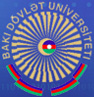## Specialties of the Faculty of Applied Mathematics and Cybernetics

• Computer Science
• IT-teacher

Master's degree

Specialty "Mathematics":

• Theory of Probability and Mathematical Statistics
• Operations Research and Systematic Analysis
• Mathematical Cybernetics
• Mathematical physics
• Optimization and optimal control
• Discrete systems
• Computational diagnostics
• Analysis of complex systems by probabilistic and statistical methods

Specialty "Computer Science":

• Software for computer systems and networks
• Informatics
• Applied mathematics
• Mathematical economic methods of optimal control
• Mathematical modeling
• Mathematical and information support of economic activity
• Application of the theory of dynamic systems to dynamic models of economic development
• Analysis of complex systems by probabilistic and statistical methods
• Economic information systems
• Information systems
• Development of mobile applications and game design
• Computer science and technology

Doctor degree
1211.01 - Differential Equations
1208.01 - Probability theory
1209.01 - Mathematical statistics
1214.01 - Dynamical systems and optimal control
3338.01 - System analysis, management and information processing
5302.01 - Econometrics